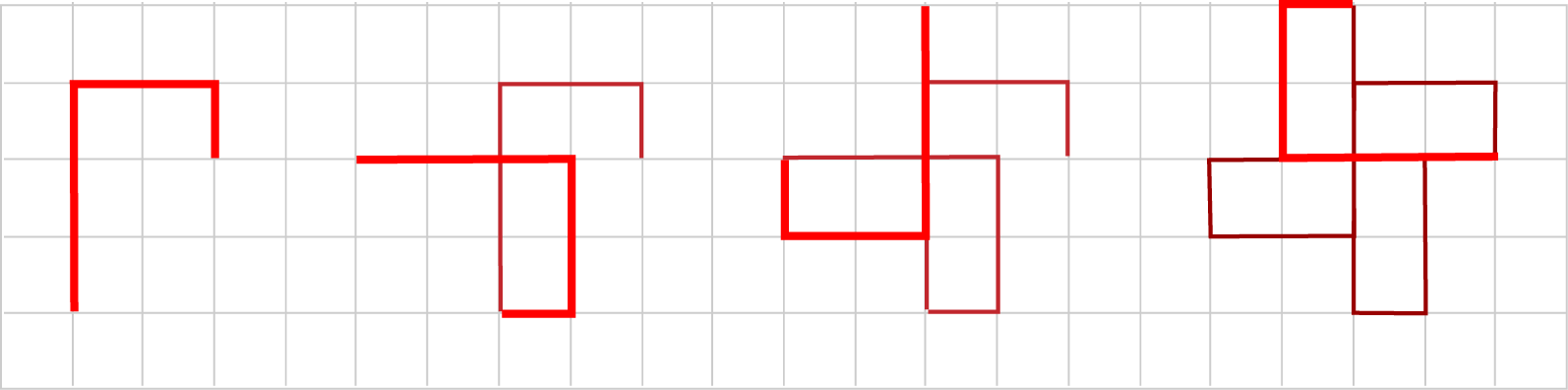# Spirolaterals

Spirolaterals are geometrical figures formed by the repetition of a simple rule. The base pattern is formed by drawing line segments of increasing length (in integer units) up to a particular size, turning a fixed angle after each segment (clockwise or anti-clockwise). Drawing continues in the same manner from the resulting position until either the figure so created returns to its starting point, or it becomes apparent that it will never do so.

For example, consider a 3 segment, 90 degree process. The figure below shows each complete cycle in the process in bright red (three line segments of lengths 1, 2 and 3, with a left hand turn of 90 degrees between each segment), and that after four cycles, the line returns to the starting point thus closing the figure.Following this process with various angles and numbers of segments yields a surprisingly rich family of figures. Various examples can be found by following the links at the bottom of this page, and the activity below lets you explore this fascinating algorithm directly.

Spirolaterals
Simple rules, complex shapes…
Use the sliders to choose the number of segments and the turning angle, and toggle the direction of individual turns using the Reversals buttons. Hit Random to view an arbitrary spirolateral. To view just a single cycle, hit the Draw One button, or Animate One to view it being drawn segment by segment. Clicking Curve/Line will cycle between line segments, and quadratic and cubic Bézier curves (where intermediate points are interpreted as control points for the curves). Press and hold on the picture to bring up a magnifier.

Segments:        Angle (degrees):

Background:   Colour: